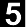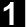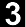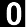Pedosphere 28(5): 764--774, 2018
ISSN 1002-0160/CN 32-1315/P
©2018 Soil Science Society of China
 Estimation of Sunflower Seed Yield Using Partial Least Squares Regression and Artificial Neural Network Models ZENG Wenzhi1, XU Chi1, Gang ZHAO2, WU Jingwei1, and HUANG Jiesheng11State Key Laboratory of Water Resources and Hydropower Engineering Science, Wuhan University(China)2Crop Science Group, Institute of Crop Science and Resource Conservation, University of Bonn, Katzenburgweg 5, Bonn 53115(Germany) ABSTRACT Statistical models can efficiently establish the relationships between crop growth and environmental conditions while explicitly quantifying uncertainties. This study aimed to test the efficiency of statistical models established using partial least squares regression (PLSR) and artificial neural network (ANN) in predicting seed yields of sunflower (Helianthus annuus). Two-year field trial data on sunflower growth under different salinity levels and nitrogen (N) application rates in the Yichang Experimental Station in Hetao Irrigation District, Inner Mongolia, China, were used to calibrate and validate the statistical models. The variable importance in projection score was calculated in order to select the sensitive crop indices for seed yield prediction. We found that when the most sensitive indices were used as inputs for seed yield estimation, the PLSR could attain a comparable accuracy (root mean square error (RMSE)=0.93 t ha-1, coefficient of determination (R2)=0.69) to that when using all measured indices (RMSE=0.81 t ha-1, R2=0.77). The ANN model outperformed the PLSR for yield prediction with different combinations of inputs of both microplots and field data. The results indicated that sunflower seed yield could be reasonably estimated by using a small number of crop characteristic indices under complex environmental conditions and management options (e.g., saline soils and N application). Since leaf area index and plant height were found to be the most sensitive crop indices for sunflower seed yield prediction, remotely sensed data and the ANN model may be joined for regional crop yield simulation. Key Words:  leaf area index,microplot experiment,plant height,remote sensing,salinization,variable importance in projection score Citation: Zeng W Z, Xu C, Zhao G, Wu J W, Huang J S. 2018. Estimation of sunflower seed yield using partial least squares regression and artificial neural network models. Pedosphere. 28(5):764-774. View Full Text